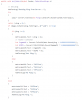# How are Ping, IGP, and GCD calculated?

Status
Not open for further replies.

#### mvrht

##### Cricket
Member
This is more of a question than a bug, but...

BnsBuddy displays 3 ping numbers
for an example:How are these numbers obtained?
What is the IP addressed used to ping?

IGP and GCD are estimations, correct? What is the formula for these estimations?

#### Endless

##### Founder
Staff member
This is more of a question than a bug, but...

BnsBuddy displays 3 ping numbers
for an example:How are these numbers obtained?
What is the IP addressed used to ping?

IGP and GCD are estimations, correct? What is the formula for these estimations?
provided from my github
as shown below
you take the ping from server, do the math 50 * 2.8333333333... = ingame ms / 50 * 1.666666666...7 = gcd ms#### mvrht

##### Cricket
Member
Thank you for the follow up.

(1)
>new Ping().Send(IP).RoundtripTime

Is IP a static variable here? What is it?

(2)
>do the math 50 * 2.8333333333... = ingame ms / 50 * 1.666666666...7 = gcd ms

Can you explain why you are multiplying by 50*2.8333 and then multiplying by 1.66666?

#### Endless

##### Founder
Staff member
Thank you for the follow up.

(1)
>new Ping().Send(IP).RoundtripTime

Is IP a static variable here? What is it?

(2)
>do the math 50 * 2.8333333333... = ingame ms / 50 * 1.666666666...7 = gcd ms

Can you explain why you are multiplying by 50*2.8333 and then multiplying by 1.66666?

1: dynamic, it calculates the time it takes to send a packet to it's destination.

2: the 50 is the current ping as shown in the image(taken for the example), 2.833... is an calculation based from my ingame ping. divided by my actual ping. So i used that to get the estimation. As for GCD, 250 was optimal for 150 ingame ms so i divided 250 by 150~ and got a number from that one to calculate the estimation.

#### mvrht

##### Cricket
Member
1: dynamic, it calculates the time it takes to send a packet to it's destination.

2: the 50 is the current ping as shown in the image(taken for the example), 2.833... is an calculation based from my ingame ping. divided by my actual ping. So i used that to get the estimation. As for GCD, 250 was optimal for 150 ingame ms so i divided 250 by 150~ and got a number from that one to calculate the estimation.

1. I was under the impression that the server always had the same IP: 64.25.35.100, because various guides always refer to it:

Is this not the case for BnsBuddy?

2. Ah, I understand. You're using your own ping as a reference. That makes sense.

Staff member

#### mvrht

##### Cricket
Member
right, of course...

#### Endless

##### Founder
Staff member
got all the info you needed?